Courses

# Syllogism MCQ 1

## 20 Questions MCQ Test Logical Reasoning for CLAT | Syllogism MCQ 1

Description
This mock test of Syllogism MCQ 1 for CLAT helps you for every CLAT entrance exam. This contains 20 Multiple Choice Questions for CLAT Syllogism MCQ 1 (mcq) to study with solutions a complete question bank. The solved questions answers in this Syllogism MCQ 1 quiz give you a good mix of easy questions and tough questions. CLAT students definitely take this Syllogism MCQ 1 exercise for a better result in the exam. You can find other Syllogism MCQ 1 extra questions, long questions & short questions for CLAT on EduRev as well by searching above.
QUESTION: 1

### In each of the questions below given four statements followed by three conclusions numbered I, II and III. You have to take the given statements to be true even if they seem to be at variance from commonly known facts. Read all the conclusions and then decide which of the given conclusions logically follows from the given statements disregarding commonly known facts.​ Q. Statements: All cards are notes. All notes are tyres. All tyres are books. All books are rods. Conclusions: I. Some rods are notes. II. Some books are tyres. III. Some tyres are cards

Solution: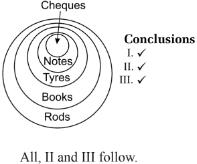QUESTION: 2

### In each of the questions below given four statements followed by three conclusions numbered I, II and III. You have to take the given statements to be true even if they seem to be at variance from commonly known facts. Read all the conclusions and then decide which of the given conclusions logically follows from the given statements disregarding commonly known facts. ​Q. ​ Statements: Some Pencils are Chairs. All Chairs are umbrellas. Some umbrellas are sticks. All sticks are caps. Conclusions: I. Some caps are umbrellas. II. Some umbrellas are sticks. III. Some sticks are pencils.

Solution: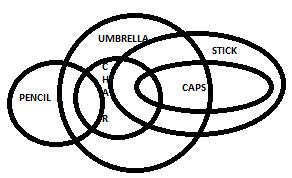QUESTION: 3

### In each of the questions below given four statements followed by three conclusions numbered I, II and III. You have to take the given statements to be true even if they seem to be at variance from commonly known facts. Read all the conclusions and then decide which of the given conclusions logically follows from the given statements disregarding commonly known facts. ​Q. ​​ Statements: Some lenses are poles. All poles are skies. Some skies are boxes. No box is a bottle. Conclusions: I. Some bottles are lens II. Some boxes are poles. III. No bottle is a lens.

Solution:

The venn diagram for this question will be as follow, the bottles can be placed at two places either along with the lens or away from the lens. So the option is E.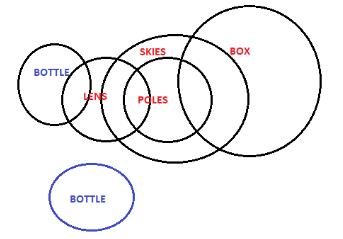QUESTION: 4

In each of the questions below given four statements followed by three conclusions numbered I, II and III. You have to take the given statements to be true even if they seem to be at variance from commonly known facts. Read all the conclusions and then decide which of the given conclusions logically follows from the given statements disregarding commonly known facts.

​Q. ​
Statements:
Some seeds are flowers.
All flowers are trees.
All trees are leaves.
Some leaves are plants

Conclusions:

I. Some plants are flowers.

II. Some leaves are seeds.

III. Some leaves are flowers.

Solution:

It is clear from the venn diagram that only conclusion 2 and 3 follows.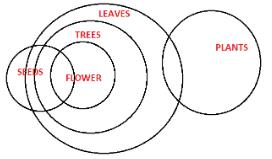QUESTION: 5

In each of the questions below are given three statements followed by three conclusions numbered I, II and III, You have to take the given statements to be true even if they seem to be at variance from the commonly known facts. Read all the conclusions and then decide which of the given conclusions logically follows from the given statements disregarding commonly known facts.

Q.
Statements
Some pens are books.
All schools are books.
Some colleges are schools.
Conclusions:

1. Some colleges are pens.
2. Some pens are schools.
3. Some colleges are books.
Solution:

Some pens are books. All schools are books.

Since the middle term 'books' is not distributed even once in the premises, so no definite conclusion follows.

Some colleges are schools. All schools are books.

Since one premise is particular, the conclusion must be particular and should not contain the middle term.

So, it follows that 'Some colleges are books'. Thus, III follows.

Some pens are books. Some colleges are books.

Since both the premises are particular, no definite conclusion can be drawn.

Hence, only III follows.

QUESTION: 6

In the questions below are given four statements followed by for conclusions numbered I, II, III and IV. You have to take given statements to be true even if they seem to be at variance from commonly known facts. Read all the conclusions and then decide which of the given conclusions logically follows from the given statements disregarding commonly known facts.

Q.
Statements:
All cups are bottles.
Some bottles are mugs.
No mug is a plate.
Some plates are tables.

Conclusions:

I. Some tables are bottles.

II. Some plates are cups

III. No table is a bottle.

IV. Some mugs are cups.

Solution:

The Venn diagram for the syllogism is as shown below: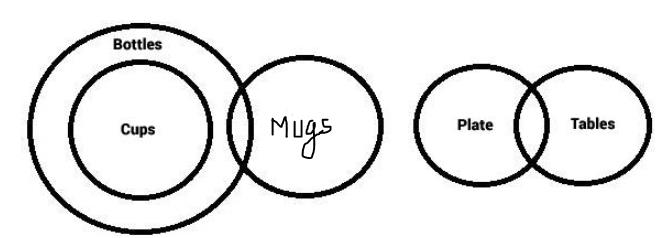The conclusions I and III are complementary in nature ie. One of the two has to be correct universally and both can't be correct at the same time. And the other conclusions are false. Hence, option (E) is the right answer.

QUESTION: 7

In the questions below are given four statements followed by for conclusions numbered I, II, III and IV. You have to take given statements to be true even if they seem to be at variance from commonly known facts. Read all the conclusions and then decide which of the given conclusions logically follows from the given statements disregarding commonly known facts.

Q.​
Statements:
All birds are horses.
All horses are tigers.
Some tigers are lions.
Some lions are donkeys.

Conclusions:

I. Some tigers are horses.

II. Some donkeys are birds.

III. Some tigers are birds.

IV. Some donkeys are horses.

Solution:

All horses are tigers so some tigers are horses. This is the conclusion I. Next All birds are horses and all horses are tigers. Therefore all birds are tigers is valid from this we can conclude some tigers are birds. that is III. therefore both I and III are valid. Option A is the correct answer.

QUESTION: 8

In the questions below are given four statements followed by for conclusions numbered I, II, III and IV. You have to the take given statements to be true even if they seem to be at variance from commonly known facts. Read all the conclusions and then decide which of the given conclusion logically follows from the given statements disregarding commonly known facts.

Q.​
Statements:
Some chairs are tables.
All tables are pots.
All pots are mats.
Some mats are buses.

Conclusions:

I. Some buses are tables.

II. Some mats are chairs.

III. No bus is tables.

IV. Some mats are tables.

Solution:

For further clarification , refer to the image.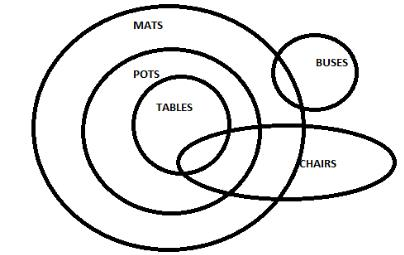QUESTION: 9

In the questions below are given four statements followed by for conclusions numbered I, II, III and IV. You have to the take given statements to be true even if they seem to be at variance from commonly known facts. Read all the conclusions and then decide which of the given conclusion logically follows from the given statements disregarding commonly known facts.

Q.​ ​​Statements:
Some Clothes are lamps.
Some flowers are lamps.
Some lamps are dresses.
All dresses are shirts.

Conclusions:

I. Some shirts are Clothes

II. Some shirts are flowers.

III. Some flowers are Clothes.

IV. Some dresses are Clothes.

Solution:

A is the correct option
lamps = some clothes, some flowers and some dresses.
All dresses are shirts, meaning, some lamps are shirts.

Hence, none of the conclusion is correct.

QUESTION: 10

In each of the questions below are given four statements followed by for conclusions numbered I, II, III and IV. You have to the take given statements to be true even if they seem to be at variance from commonly known facts. Read all the conclusions and then decide which of the given conclusion logically follows from the given statements disregarding commonly known facts.

Q.​ ​​​
Statements:
Some benches are walls.
All walls are houses.
Some houses are jungles.
All jungles are windows.

Conclusions:

I. Some windows are benches.

II. Some jungles are walls.

III. Some houses are benches.

IV. Some windows are houses.

Solution:

The correct option is C.
The venn diagram for the question is follow-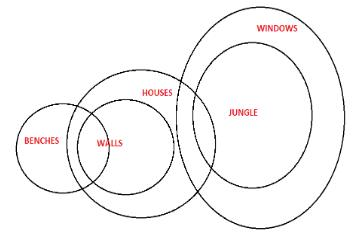QUESTION: 11

In each of the questions below are given four statements followed by for conclusions numbered I, II, III and IV. You have to take given statements to be true even if they seem to be at variance from commonly known facts. Read all the conclusions and then decide which of the given conclusion logically follows from the given statements disregarding commonly known facts.
Q.
Statements :

No cream is a lemon.

All lemons are onions.

All cream is a grapes.

Conclusion :

(i) Some grapes are not onions.

(ii) All lemons are grapes is a possibility

Solution:

The correct option is B.
From the following venn diagram it is clear that conclusion 2 follows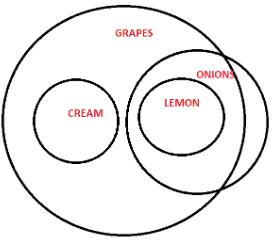QUESTION: 12

In each of the questions below are given four statements followed by for conclusions numbered I, II, III and IV. You have to the take given statements to be true even if they seem to be at variance from commonly known facts. Read all the conclusions and then decide which of the given conclusion logically follows from the given statements disregarding commonly known facts.
Q.
Statements :

Some trees are mangoes.

All mangoes are bananas.

All tomatoes are bananas.

Conclusions:

(i) All trees are tomatoes is a possibility.

(ii) No bananas are tree is a possibility.

Solution:

The answer is A because some mangoes are trees and all mangoes are bananas so some mangoes which are tree would come into bananas always hence conclusion II is not implicit.

QUESTION: 13

In each of the questions below are given four statements followed by for conclusions numbered I, II, III and IV. You have to the take given statements to be true even if they seem to be at variance from commonly known facts. Read all the conclusions and then decide which of the given conclusion logically follows from the given statements disregarding commonly known facts.
Q.
Statements :

All mobiles are cellular.

All cellular are mechanics.

All microphones are mobiles.

Conclusions :

(i) No microphone is mechanic is a possibility.

(ii) Some microphones are not cellular.

Solution:

The correct option is D.
From the venn diagram it is clear that
all microphones are possible to be mechanic and Some microphones are cellular.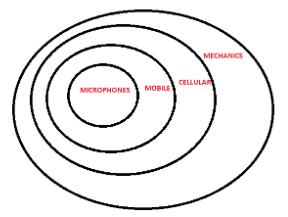QUESTION: 14

In each of the questions below are given four statements followed by for conclusions numbered I, II, III and IV. You have to the take given statements to be true even if they seem to be at variance from commonly known facts. Read all the conclusions and then decide which of the given conclusion logically follows from the given statements disregarding commonly known facts.
Q.
Statements :

All mobiles are cellular.

All cellular are mechanics.

All microphones are mobiles.

Conclusions :

(i) Some mechanics which one mobiles are also a part of microphones.

(ii) All microphones are cellular.

Solution:

The correct option is E.
It is clear from the venn diagram.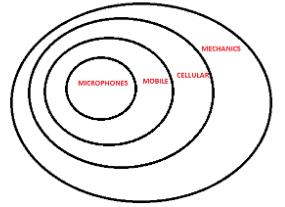QUESTION: 15

In each of the questions below are given four statements followed by for conclusions numbered I, II, III and IV. You have to the take given statements to be true even if they seem to be at variance from commonly known facts. Read all the conclusions and then decide which of the given conclusion logically follows from the given statements disregarding commonly known facts.
Q.
Statements :

All pencils are pens.

Some pens are stickers.

All stickers are numbers.

Some number are erasers.

Conclusions :

(i) Some numbers are pens.

(ii) Some numbers are stickers.

Solution: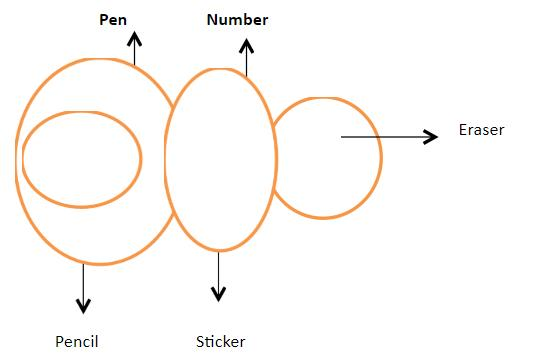QUESTION: 16

In each of the questions below are given four statements followed by for conclusions numbered I, II, III and IV. You have to the take given statements to be true even if they seem to be at variance from commonly known facts. Read all the conclusions and then decide which of the given conclusion logically follows from the given statements disregarding commonly known facts.
Q.
Statements :

All pencils are pens.

Some pens are stickers.

All stickers are numbers.

Some number are erasers.

Conclusions :

(i) Some erasers are numbers.

(ii) some pens are pencils.

Solution:

If some numbers are erasers then vice versa will also be true because maybe all erasers are not numbers but some can be. Thus the conclusion 1 is true.
Also if all pencils are pens then maybe all pens are not pencils but some will be pencils and some will be stickers.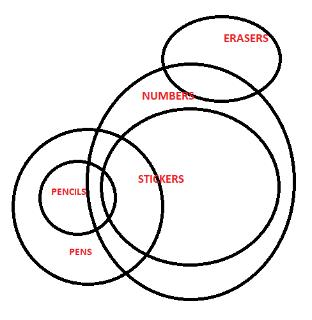QUESTION: 17

In each of the questions below are given four statements followed by for conclusions numbered I, II, III and IV. You have to the take given statements to be true even if they seem to be at variance from commonly known facts. Read all the conclusions and then decide which of the given conclusion logically follows from the given statements disregarding commonly known facts.
Q.
Statements :

All dusters are bowls.

All bowls are shoes.

No shoe is a root.

All roots are flowers.

Conclusions :

(i) No flower is a bowl.

(ii) All dusters are shoes.

Solution:

It is clear from the venn diagram that both the conclusions follow.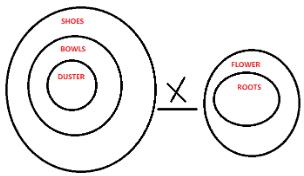QUESTION: 18

In each of the questions below are given four statements followed by for conclusions numbered I, II, III and IV. You have to the take given statements to be true even if they seem to be at variance from commonly known facts. Read all the conclusions and then decide which of the given conclusion logically follows from the given statements disregarding commonly known facts.
Q.
Statements :

All dusters are bowls.

All bowls are shoes.

No shoe is a root.

All roots are flowers.

Conclusions :

(i) No duster is a root.

(ii) All flowers are root.

Solution:

The correct option is A.
As if all roots are flowers that do not means that all flowers are roots, thus only conclusion 1 follows.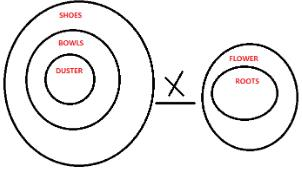QUESTION: 19

In each of the questions below are given four statements followed by for conclusions numbered I, II, III and IV. You have to the take given statements to be true even if they seem to be at variance from commonly known facts. Read all the conclusions and then decide which of the given conclusion logically follows from the given statements disregarding commonly known facts.
Q.
Statements :

Some mails are chats.

Conclusions :

(i) All mails being uploads is a possibility.

(ii) No upload is a mail.

Solution:

Some mails are chats. There is a possibility that all mails are chats. All updates are chats. So, if mails is a subset of updates, which in turn is a subset of chats, the conditions still hold true. So, conclusion I follows. Conclusion II is not necessarily true as there might be some intersection between mails and updates.

QUESTION: 20

In each of the questions below are given four statements followed by for conclusions numbered I, II, III and IV. You have to the take given statements to be true even if they seem to be at variance from commonly known facts. Read all the conclusions and then decide which of the given conclusion logically follows from the given statements disregarding commonly known facts.
Q.
Statements :

All guns are parachutes.

No parachutes is helicopter.

All helicopters are airplanes.

Conclusions :

(i) No gun is helicopter.

(ii) All guns being airplanes is a possibility.

Solution: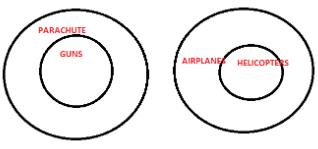There is no connection between guns and airplanes so conclusion 2 is wrong. Only conclusion 1 is correct